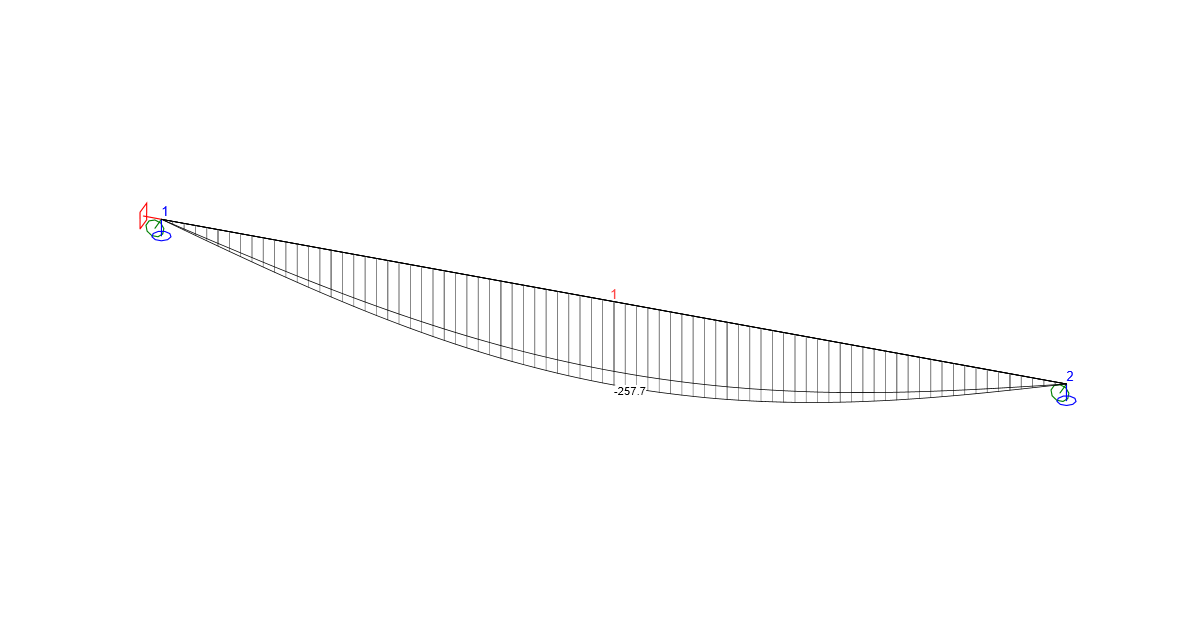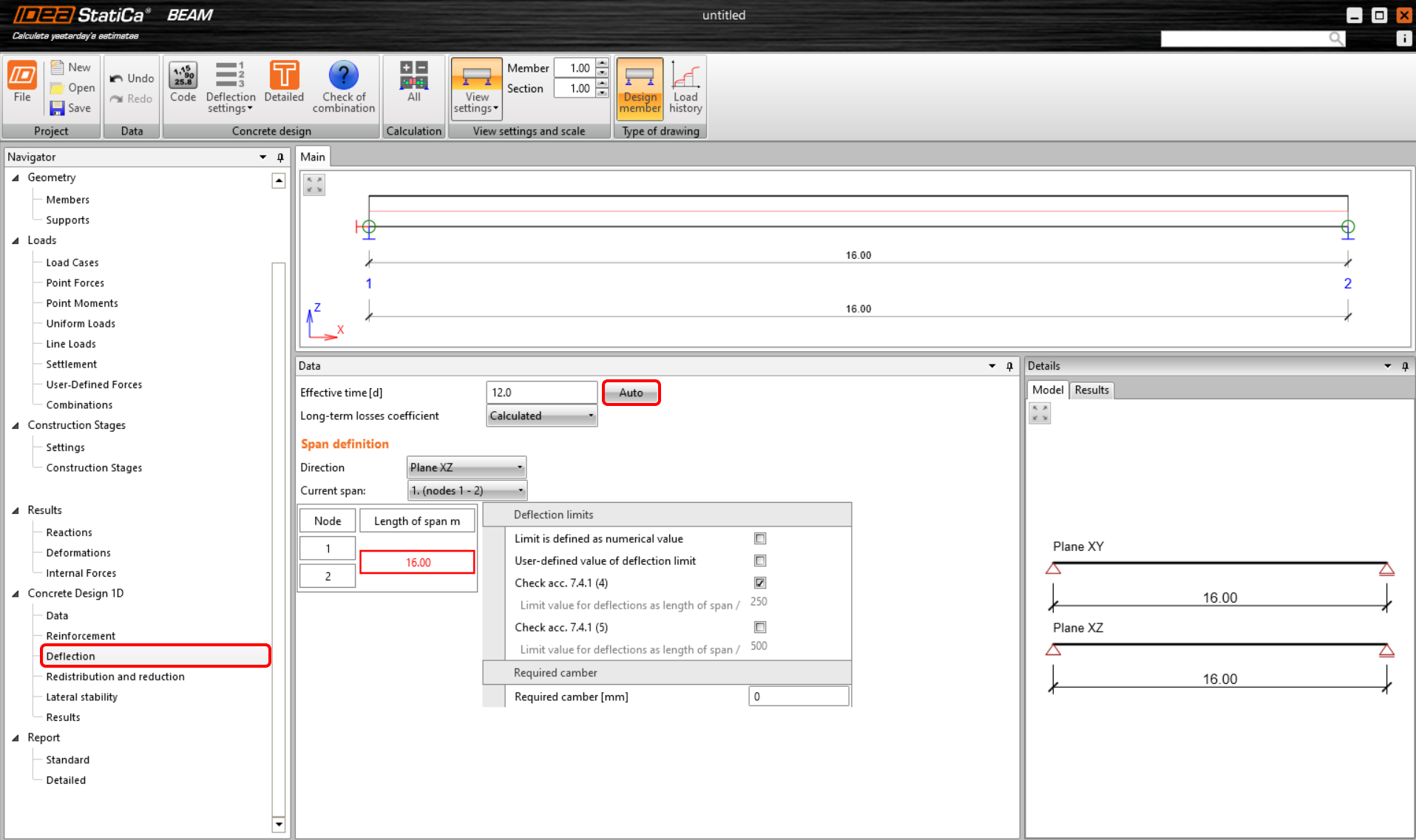### Choose language# Equivalent time for deflection evaluation

To determine the non-linear long-term deflection of staged elements, the time for the application of long-term load has to be defined. Since version 22.1.3, it is possible to calculate effective time automatically using new functionality in the Beam application.
$$The calculation is based on a linear deflection from permanent loads (or prestressing) applied in construction stages. The calculation will be performed after clicking the Auto button (the value can still be defined manually).The effective time d is calculated according to the formula:$d = \frac{{\sum\limits_{n = 1}^i {{t_i}\cdot\,{u_{st,i}}} }}{{\sum\limits_{n = 1}^i {{u_{st,i}}} }}$whereti time when the load (or prestressing) for each phase is applied ust,i the absolute value of the maximum short-term linear deflection from the applied load (or prestressing) The particular times used when the load is applied can be found in the construction stage tab, whereby the Superimposed dead load is the last phase taken into account. The used value of the linear deflection has been calculated by the software. For the construction stage in which we apply prestressing, we consider both values in the calculation - from the permanent load and from prestressing. Available in both Expert and Enhanced editions of IDEA StatiCa Concrete and Prestressing.$$